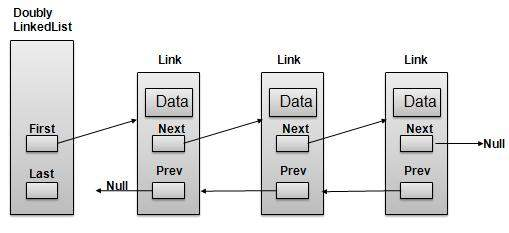# DSA using Java - Doubly Linked List

Doubly Linked List is a variation of Linked list in which navigation is possible in both ways either forward and backward easily as compared to Single Linked List. Following are important terms to understand the concepts of doubly Linked ListAs per above shown illustration, following are the important points to be considered.

• Each Link carries a data field(s) and a Link Field called next.

• Last Link carries a Link as null to mark the end of the list.

## Basic Operations

Following are the basic operations supported by an list.

• Insertion − add an element at the beginning of the list.

• Deletion − delete an element at the beginning of the list.

• Insert Last − add an element in the end of the list.

• Delete Last − delete an element from the end of the list.

• Insert After − add an element after an item of the list.

• Delete − delete an element from the list using key.

• Display forward − displaying complete list in forward manner.

• Display backward − displaying complete list in backward manner.

## Insertion Operation

Following code demonstrate insertion operation at beginning in a doubly linked list.

```//insert link at the first location
public void insertFirst(int key, int data){

if(isEmpty()){
}else {
}

//point it to old first link
//point first to new first link
}
```

## Deletion Operation

Following code demonstrate deletion operation at beginning in a doubly linked list.

```//delete link at the first location
if(first.next == null){
last = null;
}else {
first.next.prev = null;
}
first = first.next;
}
```

## Insertion at End Operation

Following code demonstrate insertion operation at last position in a doubly linked list.

```//insert link at the last location
public void insertLast(int key, int data){

if(isEmpty()){
}else {
//mark old last node as prev of new link
}

//point last to new last node
}
```

## Demo

```package com.tutorialspoint.list;

public int key;
public int data;

this.key = key;
this.data = data;
}

public void display(){
System.out.print("{"+key+","+data+"}");
}
}
```

```package com.tutorialspoint.list;

// create an empty linked list
first = null;
last = null;
}

//is list empty
public boolean isEmpty(){
return first == null;
}

//insert link at the first location
public void insertFirst(int key, int data){

if(isEmpty()){
}else {
}

//point it to old first link
//point first to new first link
}

//insert link at the last location
public void insertLast(int key, int data){

if(isEmpty()){
}else {
//mark old last node as prev of new link
}

//point last to new last node
}

//delete link at the first location
if(first.next == null){
last = null;
}else {
first.next.prev = null;
}
first = first.next;
}

//delete link at the last location
if(first.next == null){
first = null;
}else {
last.prev.next = null;
}
last = last.prev;
}

//display the list in from first to last
public void displayForward(){
//start from the beginning
//navigate till the end of the list
System.out.print("[ ");
while(current != null){
//print data
current.display();
//move to next item
current = current.next;
System.out.print(" ");
}
System.out.print(" ]");
}

//display the list from last to first
public void displayBackward(){
//start from the last
//navigate till the start of the list
System.out.print("[ ");
while(current != null){
//print data
current.display();
//move to next item
current = current.prev;
System.out.print(" ");
}
System.out.print(" ]");
}

//delete a link with given key
//if list is empty
if(first == null){
return null;
}

//navigate through list
while(current.key != key){
//if it is last node
if(current.next == null){
return null;
}else{
current = current.next;
}
}

//found a match, update the link
if(current == first) {
//change first to point to next link
first = current.next;
}else {
current.prev.next = current.next;
}

if(current == last){
//change last to point to prev link
last = current.prev;
}else {
current.next.prev = current.prev;
}
return current;
}

public boolean insertAfter(int key, int newKey, int data){
//if list is empty
if(first == null){
return false;
}

//navigate through list
while(current.key != key){
//if it is last node
if(current.next == null){
return false;
}else{
current = current.next;
}
}

if(current==last) {
}
else {
}
return true;
}
}

```

```
package com.tutorialspoint.list;

public static void main(String args[]){

list.insertFirst(1, 10);
list.insertFirst(2, 20);
list.insertFirst(3, 30);

list.insertLast(4, 1);
list.insertLast(5, 40);
list.insertLast(6, 56);

System.out.print("\nList (First to Last): ");
list.displayForward();
System.out.println("");
System.out.print("\nList (Last to first): ");
list.displayBackward();

System.out.print("\nList , after deleting first record: ");
list.deleteFirst();
list.displayForward();

System.out.print("\nList , after deleting last record: ");
list.deleteLast();
list.displayForward();

System.out.print("\nList , insert after key(4) : ");
list.insertAfter(4,7, 13);
list.displayForward();

System.out.print("\nList  , after delete key(4) : ");
list.delete(4);
list.displayForward();

}
}

```

If we compile and run the above program then it would produce following result −

```List (First to Last): [ {3,30} {2,20} {1,10} {4,1} {5,40} {6,56}  ]

List (Last to first): [ {6,56} {5,40} {4,1} {1,10} {2,20} {3,30}  ]
List (First to Last) after deleting first record: [ {2,20} {1,10} {4,1} {5,40} {6,56}  ]
List  (First to Last) after deleting last record: [ {2,20} {1,10} {4,1} {5,40}  ]
List  (First to Last) insert after key(4) : [ {2,20} {1,10} {4,1} {7,13} {5,40}  ]
List  (First to Last) after delete key(4) : [ {2,20} {1,10} {7,13} {5,40}  ]
```